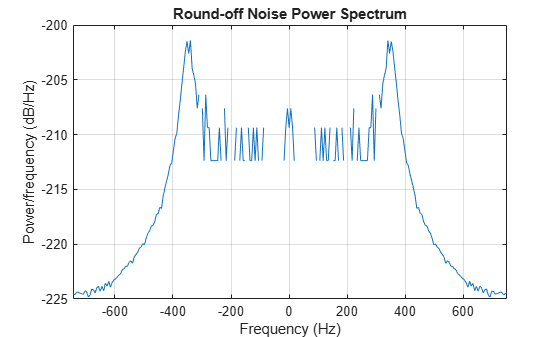# noisepsdopts

Create an options object for output noise PSD computation

## Syntax

``opts = noisepsdopts(sysobj)``

## Description

example

````opts = noisepsdopts(sysobj)` uses the current settings in the filter System object™ to create an options object that contains specified options for computing the output noise power spectral density. You can pass the `opts` object as an input argument to the `noisepsd` function.```

## Examples

collapse all

Use the `noisepsdopts` function to set options to compute the output noise PSD. `filt1` and `filt2` are lowpass filters that use different design methods. The `opts` object makes it easier to set the same conditions for the noise PSD computation in the `noisepsd` function.

`d = fdesign.lowpass`
```d = lowpass with properties: Response: 'Lowpass' Specification: 'Fp,Fst,Ap,Ast' Description: {4x1 cell} NormalizedFrequency: 1 Fpass: 0.4500 Fstop: 0.5500 Apass: 1 Astop: 60 ```
`filt1 = design(d,'butter','Systemobject',true)`
```filt1 = dsp.SOSFilter with properties: Structure: 'Direct form II' CoefficientSource: 'Property' Numerator: [13x3 double] Denominator: [13x3 double] HasScaleValues: true ScaleValues: [0.4151 0.3718 0.3374 0.3099 0.2878 0.2701 0.2558 0.2445 0.2358 0.2293 0.2248 0.2221 0.4704 1] Use get to show all properties ```
`filt2 = design(d,'cheby2','Systemobject',true)`
```filt2 = dsp.SOSFilter with properties: Structure: 'Direct form II' CoefficientSource: 'Property' Numerator: [5x3 double] Denominator: [5x3 double] HasScaleValues: true ScaleValues: [0.7003 0.5771 0.4734 0.3925 0.3466 1] Use get to show all properties ```
`opts = noisepsdopts(filt1)`
```opts = struct with fields: FreqPoints: 'All' NFFT: 512 NormalizedFrequency: true Fs: 'Normalized' SpectrumType: 'Onesided' CenterDC: false ConfLevel: 'Not Specified' ConfInterval: [] ```
```opts.NFFT = 256; % Same as set(opts,'nfft',256). opts.NormalizedFrequency = false; opts.Fs = 1.5e3; opts.CenterDC = true```
```opts = struct with fields: FreqPoints: 'All' NFFT: 256 NormalizedFrequency: false Fs: 1500 SpectrumType: 'Twosided' CenterDC: true ConfLevel: 'Not Specified' ConfInterval: [] ```

With `opts` configured as needed, use it as an input argument for the `noisepsd` function.

```npsd = noisepsd(filt1,20,opts); plot(npsd) title("Round-off Noise Power Spectrum")``````npsd = noisepsd(filt2,20,opts); plot(npsd) title("Round-off Noise Power Spectrum")```collapse all

## Output Arguments

collapse all

Options object which contains the options for computing the output noise PSD, returned as a `spectrum` object. You pass the `opts` object as an input argument to the `noisepsd` function to specify values for the input parameters.

Using `opts`, you can set the following properties for `noisepsd`:

Property Name

Default Value

Description and Valid Entries

`NFFT`

`512`

Specify the number of FFT points to use to calculate the PSD.

`NormalizedFrequency`

`true`

Determine whether to use normalized frequency. Enter a logical value of the logical `true` or `false`. Because this property is a logical value, do not enclose with single quotation marks.

`Fs`

`'Normalized'`

Specify the sampling frequency to use when you set `NormalizedFrequency` to `false`. Use any integer value greater than 1. Enter the value in Hz.

`SpectrumType`

`'Onesided'`

Specify how `noisepsd` should generate the PSD. Options are `'Onesided'` or `'Twosided'`. If you choose a two-sided computation, you can also choose `CenterDC` = `true`. Otherwise, `CenterDC` must be `false`.

• `'Onesided'` converts the type to a spectrum that is calculated over half the Nyquist interval. All properties affected by the new frequency range are adjusted automatically.

• `'Twosided'` converts the type to a spectrum that is calculated over the whole Nyquist interval. All properties affected by the new frequency range are adjusted automatically.

`CenterDC`

`false`

Shift the zero-frequency component to the center of a two-sided spectrum.

• When you set `SpectrumType` to `'Onesided'`, it is changed to `'Twosided'` and the data is converted to a two-sided spectrum.

• Setting `CenterDC` to `false` shifts the data and the frequency values in the object so that DC is in the left edge of the spectrum. This operation does not effect the `SpectrumType` property setting.

`Arithmetic``arithType`Analyze the filter System object, based on the arithmetic specified in the `arithType` input. `arithType` can be set to `'double'`, `'single'`, or `'fixed'`. The analysis tool assumes a double-precision filter when the arithmetic input is not specified and the filter System object is in an unlocked state.

## Version History

Introduced in R2011a

expand all